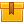## Prerequisites

Review of the lesson, Introduction to AC Components and Motors, or knowledge of AC motor components and magnetic principles is recommended.

## Description

The second lesson in the iKNOW® AC/DC Motor Theory Library, this lesson explains synchronous speed and how to calculate it. The lesson demonstrates the relationship between phased current and rotor spin and induction and its effect on a rotor. Slip and how to calculate slip using its formula are also covered.

## Objectives

Explain and be able to calculate synchronous speed. Explain induction and its effect on a rotor. Explain the relationship between phased current and rotor spin. Explain slip and know its formula.

## ContentThe second lesson in the iKNOW™ AC/DC Motor Theory Library, this lesson
explains synchronous speed and how to calculate it. The lesson
demonstrates the relationship between phased current and rotor spin and
induction and its effect on a rotor. Slip and how to calculate slip
using its formula are also covered.

•ACDCMotorTheoryAdvACMotorPrinc

0
0 Reviews